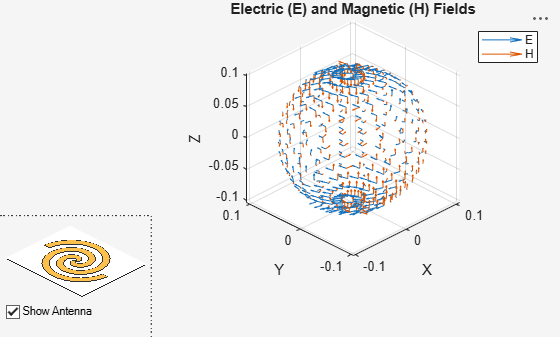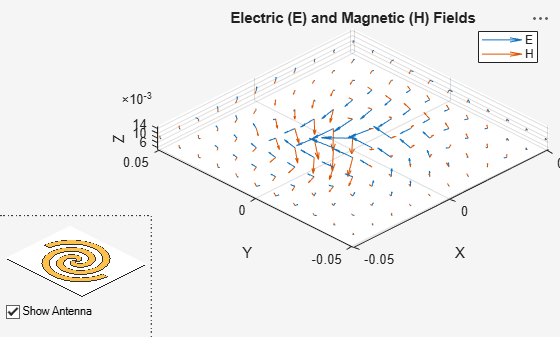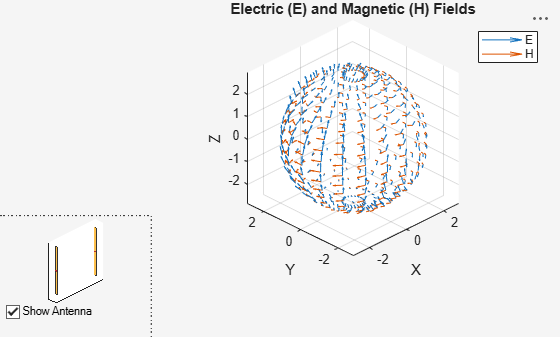Documentation

# EHfields

Electric and magnetic fields of antennas; Embedded electric and magnetic fields of antenna element in arrays

## Syntax

``````[e,h] = EHfields(object,frequency)``````
``EHfields(object,frequency)``
``````[e,h] = EHfields(object,frequency,points)``````
``EHfields(object, frequency, points)``
``EHfields(___,Name,Value)``

## Description

``````[e,h] = EHfields(object,frequency)``` calculates the x, y, and z components of electric field and magnetic field of an antenna or array object at a specified frequency.```

example

````EHfields(object,frequency)` plots the electric and magnetic field vectors at specified frequency values and at specified points in space.```

example

``````[e,h] = EHfields(object,frequency,points)``` calculates the x, y, and z components of electric field and magnetic field of an antenna or array object. These fields are calculated at specified points in space and at a specified frequency.```

example

````EHfields(object, frequency, points)` plots the electric and magnetic field vectors at specified frequency values and at specified points in space.```
````EHfields(___,Name,Value)` plots the electric and magnetic field vectors with additional options specified by one or more `Name Value` pair arguments using any of the preceding syntaxes. Use the `'ElementNumber'` and `'Termination'` property to calculate the embedded electric and magnetic fields of the antenna element in an array connected to a voltage source. The voltage source model consists of an ideal voltage source of 1 volt in series with a source impedance. The embedded pattern includes the effect of mutual coupling due to the other antenna elements in the array.```

## Examples

collapse all

Plot electric and magnetic fields of a default Archimedean spiral antenna.

```h = spiralArchimedean; EHfields(h,4e9)```Calculate electric and magnetic fields at a point 1m along the z-axis from an Archimedean spiral antenna.

```h = spiralArchimedean; [e,h] = EHfields(h,4e9,[0;0;1])```
```e = 3×1 complex 0.4137 + 0.2557i 0.3040 - 0.4084i 0.0000 + 0.0000i ```
```h = 3×1 complex -0.0008 + 0.0011i 0.0011 + 0.0007i -0.0000 - 0.0000i ```

Create an Archimedean spiral antenna. Plot electric and magnetic field vector at the z = 1cm plane from the antenna.

`h = spiralArchimedean;`

Define points on a rectangular grid in the X-Y plane.

`[X,Y] = meshgrid(-.05:.01:.05,-.05:.01:.05);`

`p = [X(:)';Y(:)';.01*ones(1,prod(size(X)))];`

Plot electric and magnetic field vector at the z = 1cm plane. from the antenna

`EHfields (h,4e9,p)`Plot the embedded vector fields of a linear array when the first element is excited and all the other antenna elements are terminated using 50-ohm resistance.

```l = linearArray; EHfields(l, 70e6, 'ElementNumber', 1, 'Termination', 50);```## Input Arguments

collapse all

Antenna or array object, specified as a scalar handle.

Example: `h = spiralArchimedean`

Data Types: `function_handle`

Frequency used to calculate electric and magnetic fields, specified as a scalar in Hz.

Example: 70e6

Data Types: `double`

Cartesian coordinates of points in space, specified as a 3-by-p complex matrix. p is the number of points at which to calculate the `E-H ` field.

Example: `[0;0;1]`

Data Types: `double`

### Name-Value Pair Arguments

Example: `'ScaleFields',[2 0.5]` specifies scalar values of the electric and magnetic fields

Specify optional comma-separated pairs of `Name,Value` arguments. `Name` is the argument name and `Value` the corresponding value. `Name` must appear inside single quotes (`''`). You can specify several name and value pair arguments in any order as `Name1,Value1,...,NameN,ValueN`.

Value by which to scale the electric and magnetic fields, specified as the comma-separated pair consisting of `'ScaleFields'` and a two-element vector. The first element scales the E field and the second element scales the H-field. A value of `2` doubles the relative length of either field. A value of `0.5` to halves the length of either field. A value of `0` plots either field without automatic scaling.

Example: `'ScaleFields'`,`[2 0.5]`

Data Types: `double`

Field to display, specified as the comma-separated pair consisting of `'ViewField'` and a text input. `'E'` displays the electric field and `'H'` displays the magnetic field.

Example: `'ViewField'`, `'E'`

Data Types: `char`

Antenna element in array, specified as the comma-separated pair consisting of `'ElementNumber'` and scalar This antenna element is connected to the voltage source.

### Note

Use this property to calculate the embedded pattern of an array.

Example: `'ElementNumber'`,1

Data Types: `double`

Impedance value for array element termination, specified as the comma-separated pair consisting of `'Termination'` and scalar. The impedance value terminates other antenna elements of an array while calculating the embedded pattern of the antenna connected to the voltage source.

### Note

Use this property to calculate the embedded pattern of an array.

Example: `'Termination'`,40

Data Types: `double`

## Output Arguments

collapse all

x, y, z components of electrical field, returned as 3-by-p complex matrix in V/m. The dimension p is the Cartesian coordinates of points in space.

x, y, z components of magnetic field, returned as a 3-by-p complex matrix in H/m. The dimension p is the Cartesian coordinates of points in space.